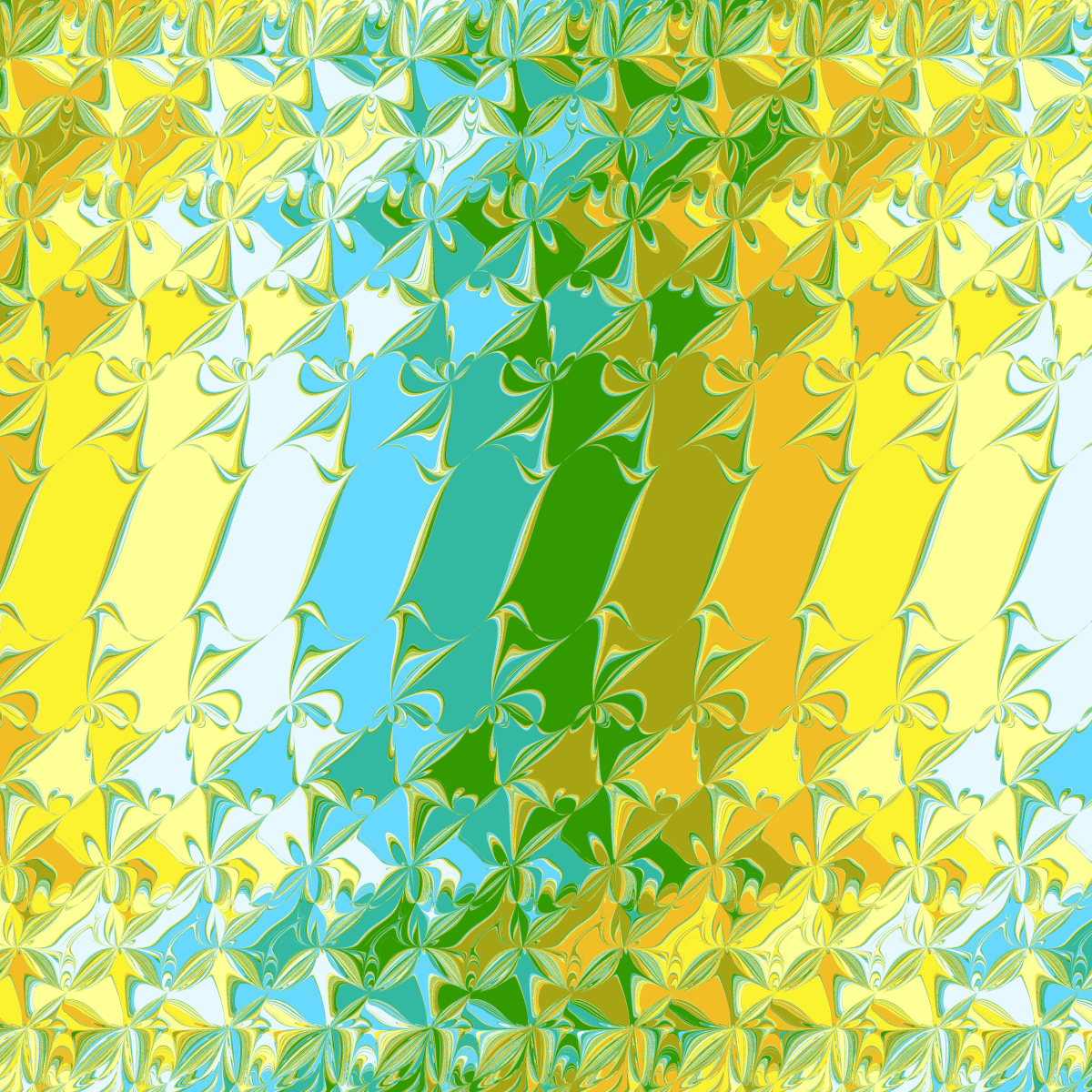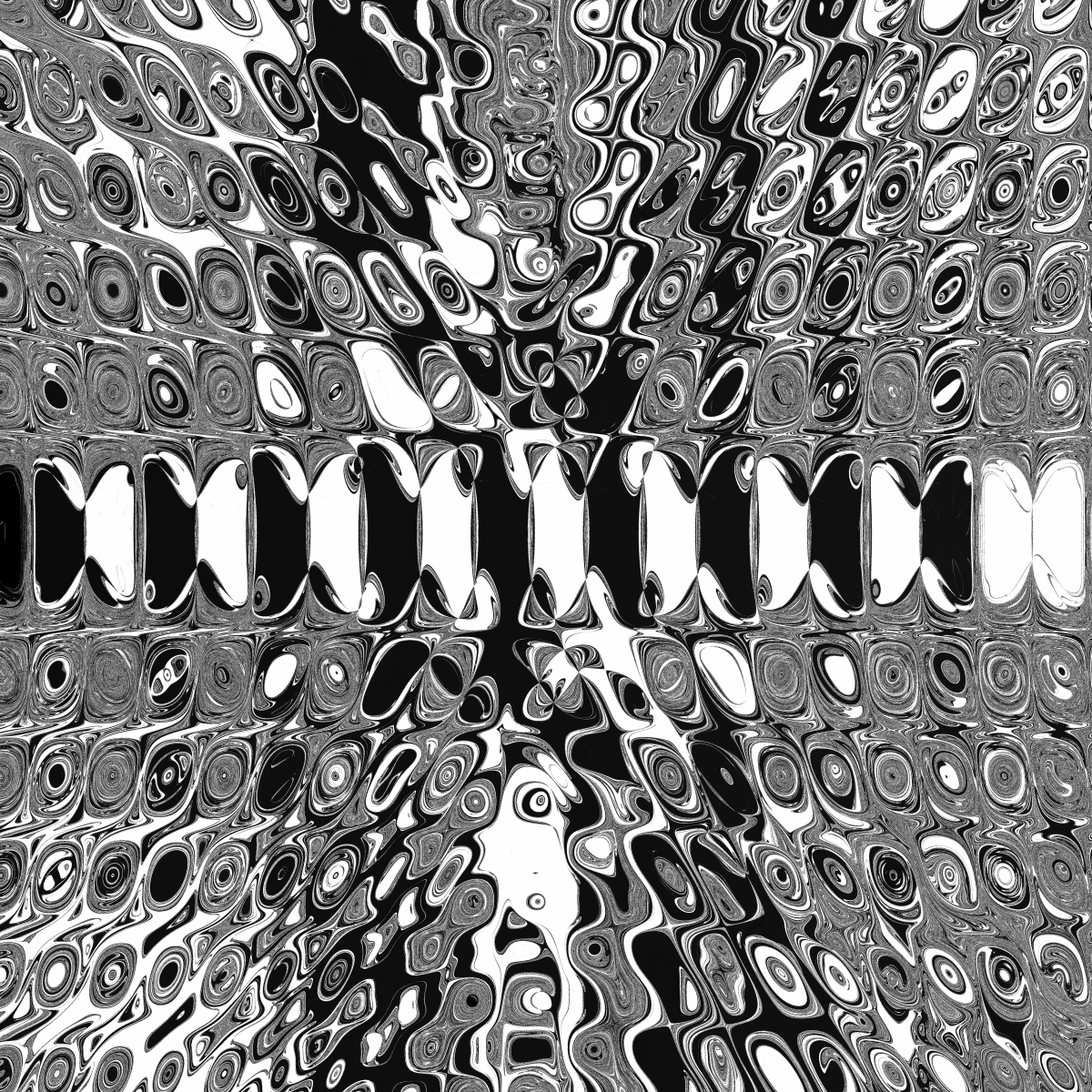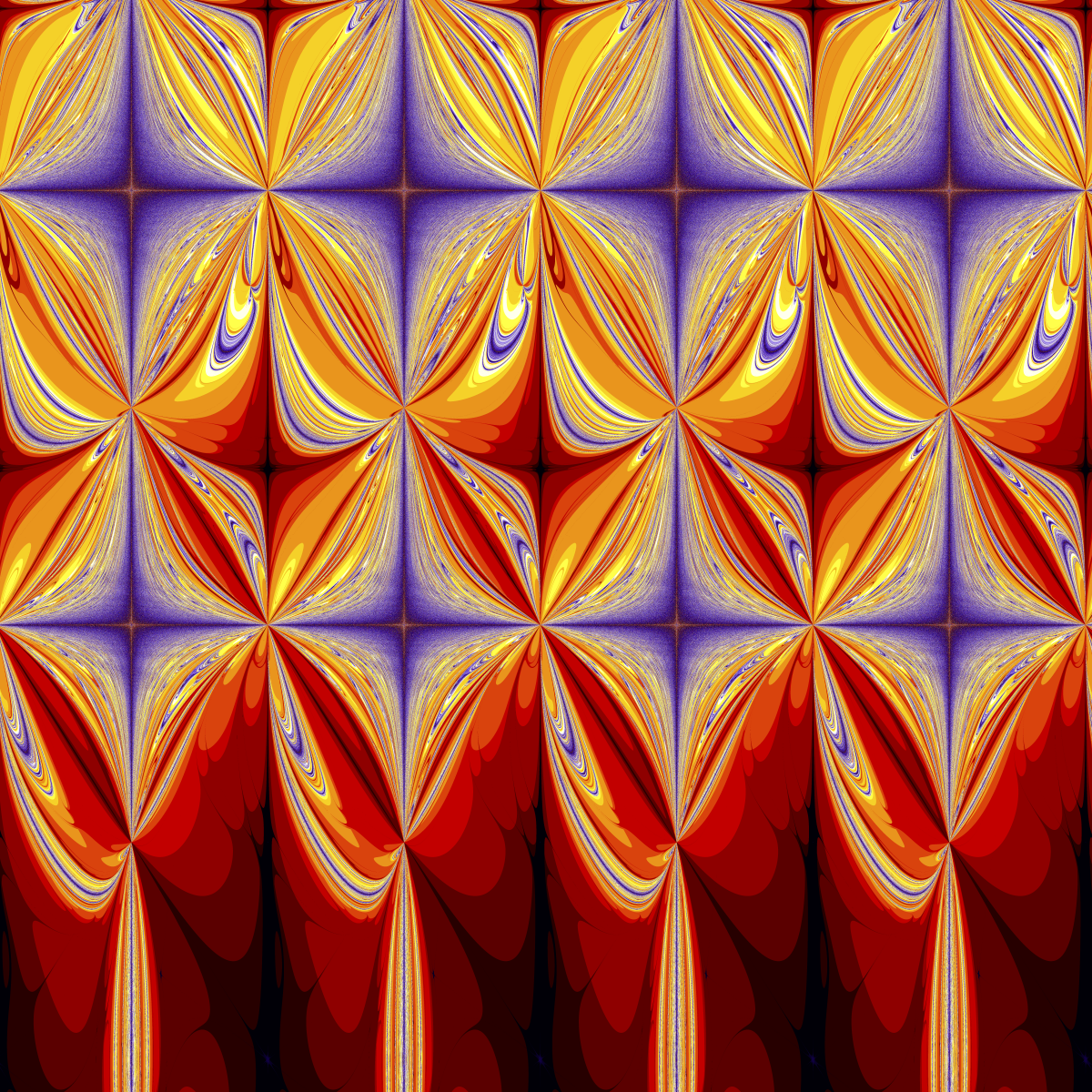### Convergence domains of the iterated parabolic approximation methodConvergence domains for the minimization of sin(x) using parabolic approximation method. Horizontal coordinate is position of the middle initial point, vertical - distance between points. The above image is a fractal, derived from iteration of the successive parabolic interpolation method, applied to searching extrema of sin(x). Wikipedia describes this method as following:
Successive parabolic interpolation is a technique for finding the extremum (minimum or maximum) of a continuous unimodal function by successively fitting parabolas (polynomials of degree two) to the function at three unique points, and at each iteration replacing the "oldest" point with the extremum of the fitted parabola.First step of the algorithm, x4 is the new point.

This method is guaranteed to converge, if applied to an unimodal function. However, the same scheme usually converges well even if applied to a function with several extrema, such as sin(x). Of course, it is only able to find one extremum among all. Which of them will be found is determined by the initial conditions. But how to determine, which initial point converge to which extremum?
Parabolic interpolation  method requires 3 initial points, thus the whole space of initial conditions is 3-dimensional. Sets of the initial points converging to the same extremum, are basins of attraction of this algorithm. And they are exhibiting nice fractal structure. The picture at the top shows these basins, each painted in different color. Since the parameter space is 3-dimensional, the whole structure is also a 3-dimensional body. To simplify it, I am using initial conditions where 3rd point is located between first two:
[x + y, x - y, x],
where x, y are coordinates on the image plane. This is equivalent to intersecting the parameter space with a 2-dimensional plane.

### More pictures

Top picture, colorized differently. If an initial point converges to maximum, it is colored white, otherwise it is black.

Enlarged fragment of the original pictureEnlarged fragment
Basins for the more complex function sin(x)+x2/100.Convergence domains for sin(x)+x^2/100
Enlarged fragment.

### Plotting number of iterations

It is also possible to assign colors according to the number of steps. In the following image, colors are starting from black (low number of steps), then go from red to blue as the number of iterations raises, and then repeats. it can be clearly seen, that when all 3 initial points are close to the extremum, method converges fast, and when it is near to the border between 2 extremums, convergence is much slower.Number of iterations

### Basin and its properties

And finally, image of a single basin (corresponding to a maximum x=π/2). All initial points colored white will converge to the same maximum.Single domain
Interesting property of this image: shifted horizontally by $\frac{\pi k}2$ where $k \in \mathbbb{Z}$, it does not overlap itself. This follows from periodicity of sin(x). The following animation shows shift by $\pi/2$.

Conclusion? In general case, correspondence between initial points and solutions, they converge to, can be surprisingly complex.
Another (and more traditional) sample of a fractal produced by the converging iterative procedure is the Newton fractal.